# Vaughan identity

In 1937, I.M. Vinogradov [a9] proved the odd case of the Goldbach conjecture (cf. also Goldbach problem); i.e., he proved that every sufficiently large odd number can be written as a sum of three prime numbers (cf. also Vinogradov method). The essential new element of his proof was a non-trivial estimate for an exponential sum involving prime numbers (cf. also Exponential sum estimates). Let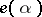denoteand let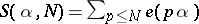, whereruns over the prime numbers. By simply observing that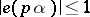for all,and using the prime number theorem (cf. de la Vallée-Poussin theorem), one immediately sees that. Vinogradov was able to improve on this estimate on the "minor arcs" ; in other words, he obtained a better estimate for those values ofthat could not be well approximated by a rational number with a small denominator. Vinogradov's estimate used the sieve of Eratosthenes (cf. also Eratosthenes, sieve of; Sieve method) to decompose the suminto subsums of the form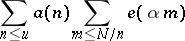and of the form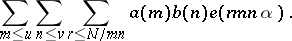The sums have become known as sums of type I and type II, respectively.

Vinogradov's method is quite powerful and can be adapted to general sums of the form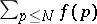. However, the technical details of his method are formidable and, consequently, the method was neither widely used nor widely understood. In 1977, R.C. Vaughan [a6] found a much simpler approach to sums over prime numbers.

Vaughan's identity is most easily understood in the context of Dirichlet series. Suppose that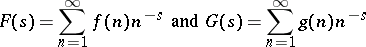are both absolutely convergent in the half-plane. Thenin this same half-plane. One of the simplest and most useful Dirichlet series is the Riemann zeta-function (cf. also Zeta-function), which is defined asfor complex numberswith real part exceeding. The Euler product formula states that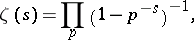where the product is over all prime numbers. Taking the reciprocal of the Euler product, one sees thatwhere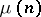is the Möbius function defined byBy looking at the coefficients of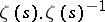, one obtains the useful identityBy taking the logarithmic derivative of the Euler product formula, one sees thatwhere the coefficientsare defined as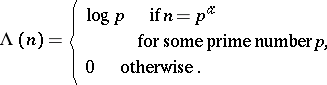This is the Mangoldt function. By computing the product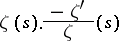in two different ways, one sees that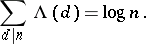For technical reasons, it is often simpler to work with sums of the form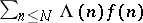than with sums of the form, and estimates for the latter sum can usually be easily derived from estimates for the former.

Let,be arbitrary real numbers, both exceeding, and defineThus,andare partial sums of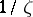and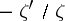respectively. In particular,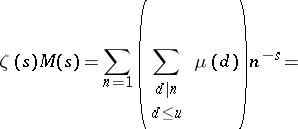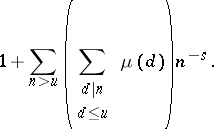Now consider the Dirichlet series identity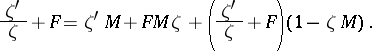Comparing coefficients of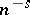on both sides of the equation, one sees that if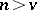, then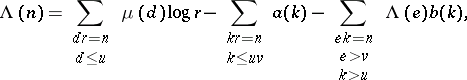where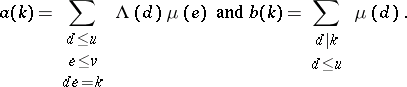If one multiplies this equation by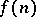and sums over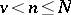, one obtains the Vaughan identity: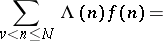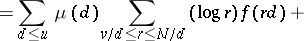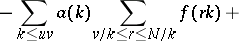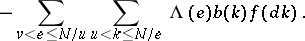In general, the first and second sums can be treated as type-I sums, and the third sum can be treated as a type-II sum. The logarithm factor in the first sum is easily finessed with partial summation. In some applications, it is useful to divide the second sum into subsums with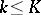and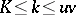, where the first subsum is treated as type-I and the second subsum as type-II.

For a brief and very accessible account of how Vaughan's identity is applied, see Vaughan's original article [a6]. There, he proves that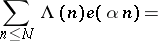whenever. Another self-contained account of this can be found in [a1].

There are many applications of Vaughan's identity in the literature. Vaughan [a7] used it to obtain new estimates on the distribution of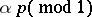, and he also used it to give an elegant proof of the Bombieri–Vinogradov theorem on prime numbers in arithmetic progressions [a8]. H.L. Montgomery and Vaughan [a5] obtained a new estimate for the error term in the formula for the number of square-free integers up to, conditional on the Riemann hypothesis (cf. Riemann hypotheses). This requires a slightly different form of Vaughan's identity. In this case, let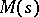be as before, but take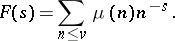From the equation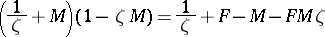one can obtain an identity for sums of the form. D.R. Heath-Brown and S.J. Patterson [a3] used Vaughan's identity to prove a long-standing conjecture of E. Kummer about distribution of cubic Gauss sums (cf. also Kummer hypothesis; Gauss sum). Heath-Brown [a2] developed a more general and more flexible version of Vaughan's identity, and G. Harman [a4] has developed an alternative treatment that returns to Vinogradov's original idea of using the sieve of Eratosthenes (cf. also Eratosthenes, sieve of).

How to Cite This Entry:
Vaughan identity. S.W. Graham (originator), Encyclopedia of Mathematics. URL: http://encyclopediaofmath.org/index.php?title=Vaughan_identity&oldid=17846
This text originally appeared in Encyclopedia of Mathematics - ISBN 1402006098